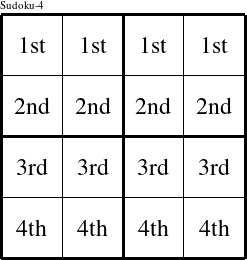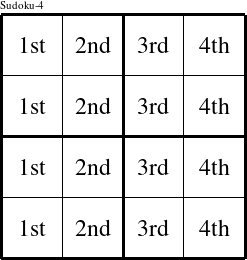#### Printable version

Detailed instructions for working a group puzzle of type 'Sudoku-4'

The 4 elements in each group of this type of puzzle are: 1, 2, 3, and 4.

Every group in the puzzle must contain exactly this set of elements.

####In a Sudoku-4 puzzle, each row is a group numbered as shown in the figure above.

####In a Sudoku-4 puzzle, each column is a group numbered as shown in the figure above.

####In a Sudoku-4 puzzle, each 2x2 square is a group numbered as shown in the figure above.